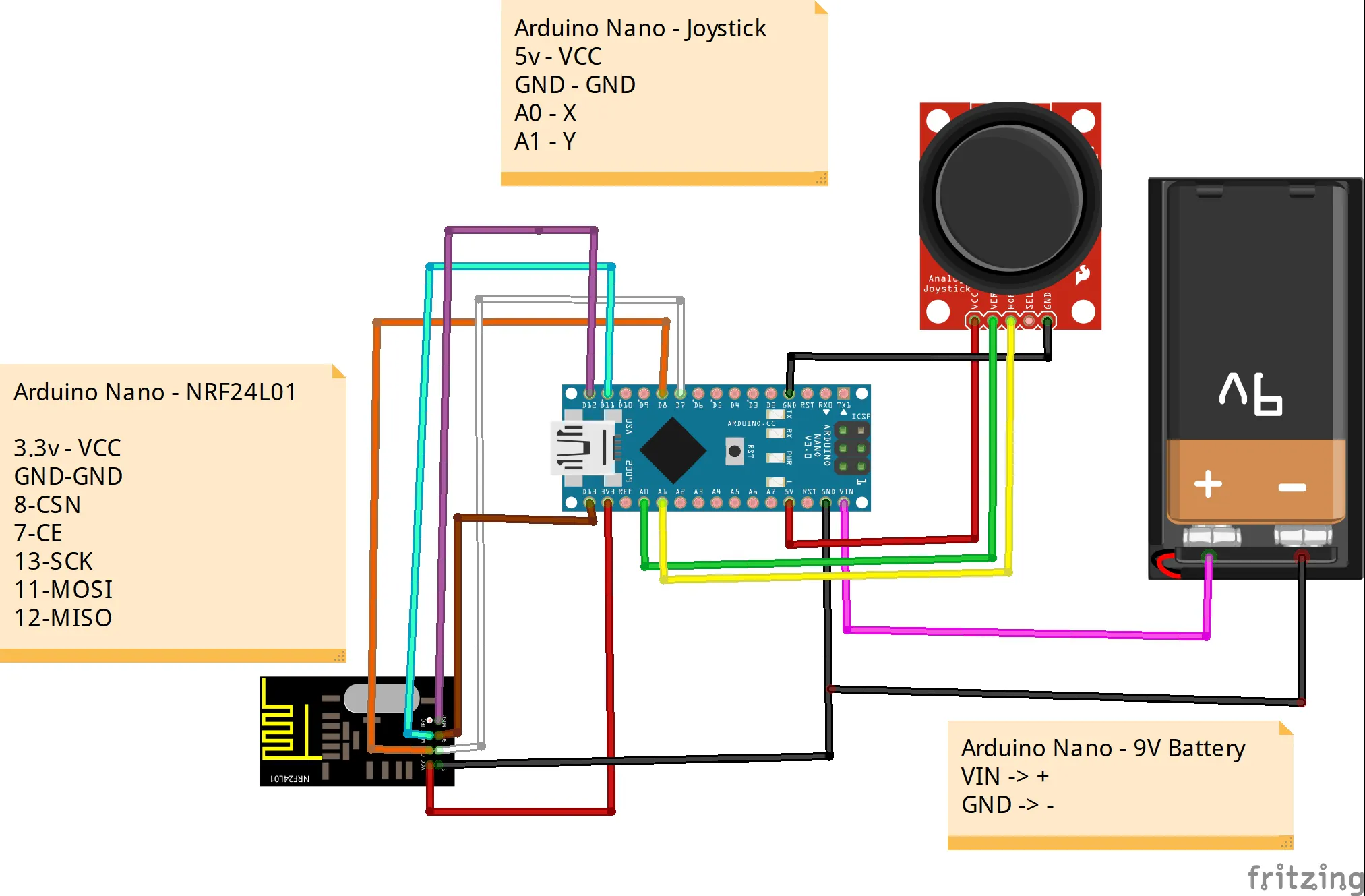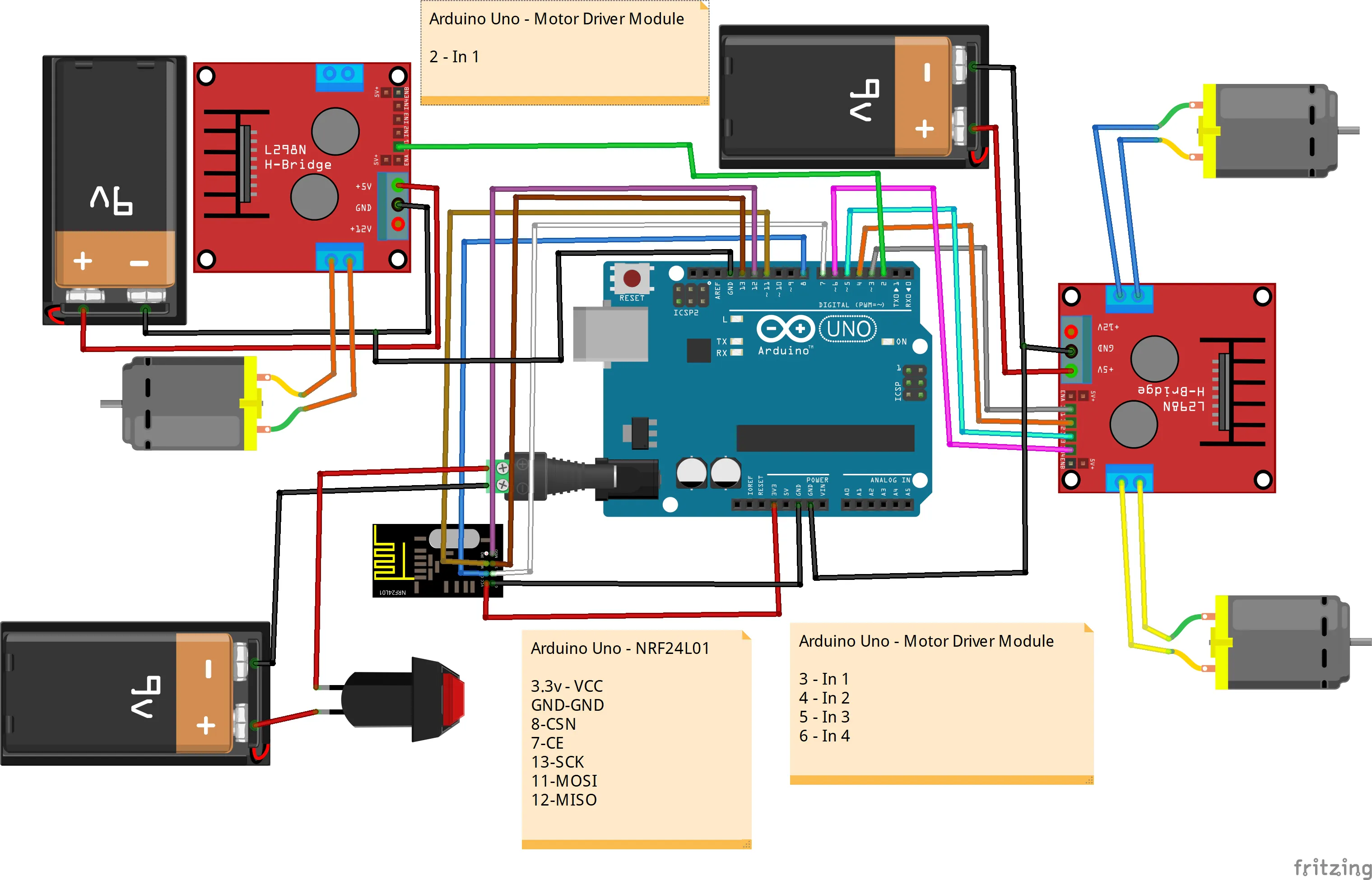# How to Build a Battlebot With Arduino and Cardboard

Build a battlebot from cardboard using Arduino.

BeginnerShowcase (no instructions)12 hours1,398

## Things used in this project

### Hardware componentsArduino UNO & Genuino UNO
×1Arduino Nano R3
×1

## Schematics

### controller_jZZRZOzlfA.jpg### Battlebot## Code

### Battlebot

Arduino
```#include <SPI.h>      //SPI library for communication with the nRF24L01+
#include "RF24.h"  //The main library of the nRF24L01+
#include "Wire.h"

RF24 radio (8, 9); // CE,CSN

//Create a pipe addresses for  communication
const uint64_t pipe = 0xE8E8F0F0E1LL;

struct package
{
int X = 1;
int Y = 1;
};

typedef struct package Package;
Package pos;

int buttonState;
int spinnerState = false ;
int motora_1 = 3;
int motora_2 = 4;
int motorb_1 = 5;
int motorb_2 = 6;
int spinner = 2;

void setup()
{
Serial.begin(9600);
pinMode(motora_1, OUTPUT);
pinMode(motora_2, OUTPUT);
pinMode(motorb_1, OUTPUT);
pinMode(motorb_2, OUTPUT);
pinMode(spinner, OUTPUT);
digitalWrite(spinner, LOW);
delay(100);
}

void loop()
{

Serial.print("X:");
Serial.print(pos.X);
Serial.print("      Y");
Serial.print(pos.Y);
Serial.print("      buttonState");
Serial.println(buttonState);
}

int xAxis = pos.X;
int yAxis = pos.Y;
int forward = map(yAxis, 570, 1024, 0, 255);
int backward = map(yAxis, 480, 0, 0, 255);
int right = map(xAxis, 570, 1024, 0, 255);
int left = map(xAxis, 480, 0, 0, 255);

if (yAxis > 570) {          // Move Forward
// Set Motor A forward
analogWrite(motora_1, forward);
analogWrite(motora_2, 0);
// Set Motor B forward
analogWrite(motorb_1, forward);
analogWrite(motorb_2, 0);
} else if (yAxis < 480) {                     //Move Backward
// Set Motor A backward
analogWrite(motora_1, 0);
analogWrite(motora_2, backward);
// Set Motor B backward
analogWrite(motorb_1, 0);
analogWrite(motorb_2, backward);
} else if (xAxis > 570) {                     // Move Right
// Set Motor A forward
analogWrite(motora_1, right);
analogWrite(motora_2, 0);
// Set Motor B stop
analogWrite(motorb_1, 0);
analogWrite(motorb_2, 0);
} else if (xAxis < 480) {                     // Move Left
// Set Motor A stop
analogWrite(motora_1, 0);
analogWrite(motora_2, 0);
// Set Motor B foward
analogWrite(motorb_1, left);
analogWrite(motorb_2, 0);
} else  {     //stop
// Set Motor A stop
analogWrite(motora_1, 0);
analogWrite(motora_2, 0);
// Set Motor B stop
analogWrite(motorb_1, 0);
analogWrite(motorb_2, 0);
}

if (buttonState == 0 ) {
spinnerState = ! spinnerState;
delay(50);
}

if (spinnerState == true) {
digitalWrite(spinner, HIGH);    //turn On spinner
delay(100);
} else {
digitalWrite(spinner, LOW);      //turn Off spinner
delay(100);
}

}
```

### Controller

Arduino
```/* YOU HAVE TO INSTALL THE RF24 LIBRARY BEFORE UPLOADING THE CODE
https://github.com/tmrh20/RF24/
*/

#include <SPI.h>        //SPI library for communicate with the nRF24L01+
#include "RF24.h"       //The main library of the nRF24L01+

//Create a pipe addresses for  communication
const uint64_t pipe = 0xE8E8F0F0E1LL;

struct package
{
int X=1;
int Y=1;
};

typedef struct package Package;
Package pos;

int xPin = A0;
int yPin = A1;

int buttonPin = 3;
int buttonState;

void setup() {
Serial.begin(9600);
pinMode(buttonPin, INPUT_PULLUP); // Activate pull-up resistor on the push button pin

}

void loop() {

// X value
Serial.print("X:");
Serial.print(pos.X);
Serial.print("    Y");
Serial.print(pos.Y);
Serial.print("    button");
Serial.println(buttonState);

radio.write(&pos, sizeof(pos)); // Send data  to the other NRF24L01 module